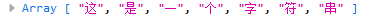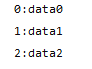# ES6学习笔记之字符串、数组、对象、函数新增知识点实例分析

## 1、模板字符串

``````
let str=`
<!DOCTYPE html>
<html lang="en">
<body>
<div>
<p class="pClass">格式化字符串</p>
</div>
</body>
</html>
`;
console.log(str);

``````

## 2、转化为数组

Array.form将伪数组、集合转化为数组

``````
let allLi=document.querySelectorAll('li');
console.log(Array.isArray(allLi));//输出false,allLi不是个数组
let arr=Array.from(allLi);
console.log(Array.isArray(arr));//输出true，arr被转化为数组

``````

Array.of将元素构建为数组

``````
let arrayOf=Array.of('str',2,{}); //创建由字符串、数字、对象构成的数组
let arr=Array.of(7); //数组包含一个元素7
let arr=Array(7); //数组包含7个空元素

``````

## 3、类的封装

``````
class Person{
constructor(name,age){ //Person类的构造函数
this.name=name;
this.age=age;
}

print(){ //类函数输出
console.log("My name is "+this.name+",I'm "+this.age+" years.");
}
}
let p=new Person('tony',15);
p.print();

``````

## 4、对象的使用

``````
let name='tony';
let age=15;
let person={
name,
age
}
console.log(person);

``````

Object { name: "tony", age: 15 }

Object.assign()方法实现对象合并，参数为：合并目标，合并源1，合并源2...

``````
let obj1={'name':'tony'};
let obj2={'age':15};
let obj3={'sex':'男'};
let obj={};
Object.assign(obj,obj1,obj2,obj3); //将后面的对象并到第一个对象
console.log(obj);

``````

Object { name: "tony", age: 15, sex: "男" }

## 5、延展操作符...

``````
let str="这是一个字符串";
let arr=[...str];
console.log(arr);

``````## 6、函数Rest参数

``````
function sum(name,...num) {
console.log(name);
let res=0;
for (let value of num){ //将后面未知个数的参数当作数组num遍历
res+=value;
}
return res;
}
console.log(sum('tony',10,2,3));

``````

## 7、箭头函数

1、当只有一个参数时，可以写为参数 => 表达式，例如使用map函数遍历一个数组，使每个元素乘2：

``````
var arr = [1, 4, 9, 16];
const map1 = arr.map(x => x * 2); //利用map()遍历数组，传入一个参数当作x，并返回x*2

``````

map中的函数以x为参数遍历每个arr中的元素，*2之后自动返回，形成map1数组

2、当有多个参数时，需要给参数加括号：() =>表达式，例如遍历数组输出索引与值：

``````
let arr=['data0','data1','data2'];
arr.forEach((value,index)=> //通过forEach遍历数组，传入两个参数value与index
console.log(index+':'+value)
);

``````3、当有多行表达式时，要用{}将函数包含成一块：() =>{}，并且使用块语句时，函数不会自动返回值，需要使用return将值返回。

4、JavaScript的{}也可以表示一个对象，当使用箭头函数返回对象时，为了与函数体区别需要将对象用()包含起来：() =>({})，否则会报错，例如在react中使用setState方法，需要返回一个对象:

``````
this.setState((prevState) =>({
flag: !prevState.flag
}))

``````

## 8、Promise函数

``````
//定义promise函数
let checkLog=function (flag) {
return new Promise(function(resolve,reject){
if(flag){ //如果判定条件为真，执行resolve函数
resolve({ //其括号内的参数作为结果返回
status:1,
info:"登陆成功"
})
}else{ //判定为假执行reject函数
reject({
status:0,
info:"登陆失败"
}
)
}
})
};
//使用promise函数
checkLog(false).then(res=>{ //根据promise返回的不同值分别执行then或catch
console.log(`成功信息：\${res.info}`);
}).catch(err=>{
console.log(`错误信息：\${err.info}`);
})

``````